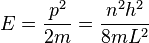# Particle in an infinite potential well

## Homework Statement

The nth energy level for a particle of mass m confined in an infinite potential well is given by:where L is the width of the well and h is Planck’s constant. Assuming that the uncertainty in the particle’s momentum is equal to the momentum itself, show that the uncertainty in the particle’s position is less than the width of the well by a factor of n.

## Homework Equations

Uncertainty principle?

## The Attempt at a Solution

I don't really know where to start. Help!

Last edited:

I have an idea of what i'm doing now, although i've got my answer as change in x <= L/(2*pi*n) instead of L/n which seems to be what the question expects.

My method was to sub in change in P = p into the uncertainty principle, then sub that into the equation given. Then i just rearranged. What am i doing wrong?

I'm also assuming all the values are positive so the less than sign doesn't have to be flipped?

Last edited:
Redbelly98
Staff Emeritus